Function Repository Resource:

# Tetration

Compute the order-k tetration of a number

 ResourceFunction["Tetration"][a,k] computes the order-k tetration of the number a.

## Details and Options

ResourceFunction["Tetration"][a,Infinity] gives a finite value if and only if a is in the convergence interval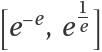.
ResourceFunction["Tetration"][a,Infinity] diverges when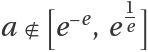.
k must be a non-negative integer or Infinity.
a must be a number different from 0.

## Examples

### Basic Examples (4)

Tetration of 2 with order 3:

 In:=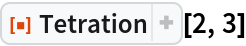Out=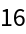Symbolic tetration:

 In:=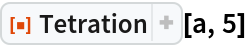Out=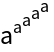Infinite tetration of a real number:

 In:=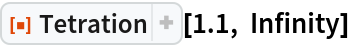Out=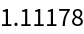Infinite tetration of :

 In:=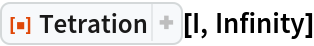Out=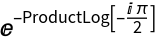In:=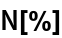Out=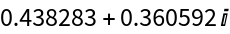### Scope (3)

Compute infinite tetrations of real numbers inside the convergence interval:

 In:=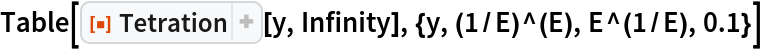Out=Compute tetrations of real numbers within the divergence interval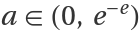:

 In:=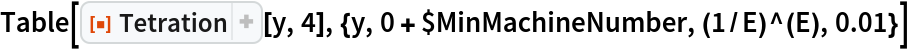Out=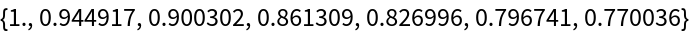Compute tetrations of real numbers within the divergence interval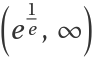:

 In:=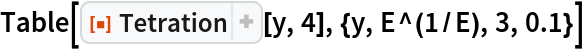Out=### Properties and Relations (5)

Behavior of the tetration of real numbers inside the divergence interval:

 In:=Out=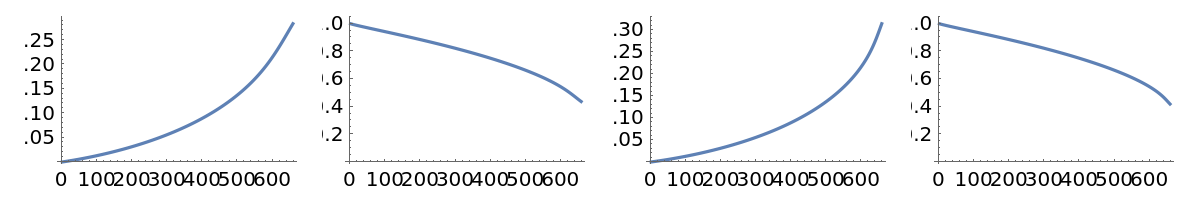Behavior of infinite tetration of real numbers inside the convergence interval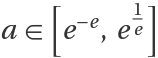:

 In:=Out=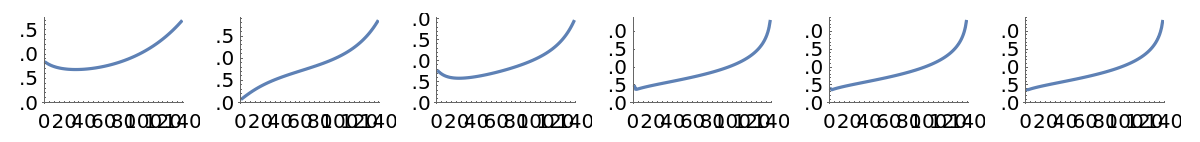Evolution and stabilization of the tetration curve inside the convergence interval for increasing k in a single plot:

 In:=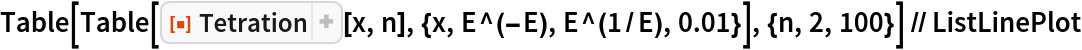Out=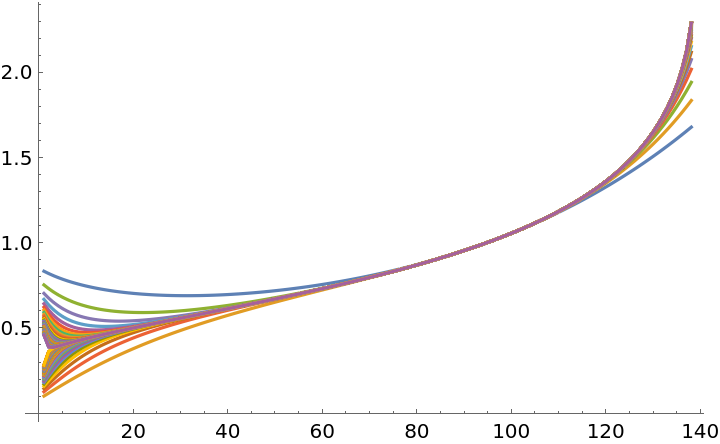Tetration convergence curve:

 In:=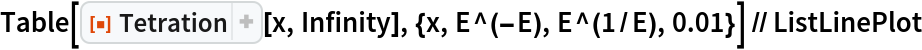Out=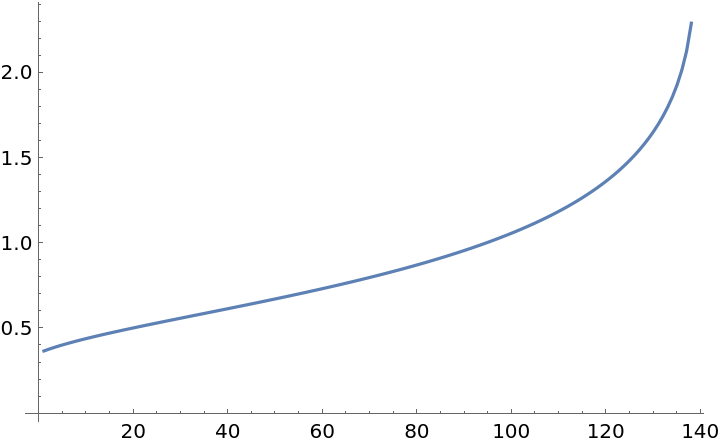Behavior of the tetration of real numbers within the divergence interval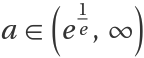:

 In:=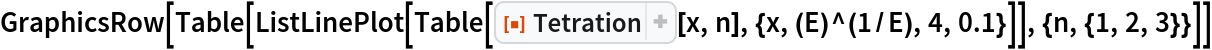Out=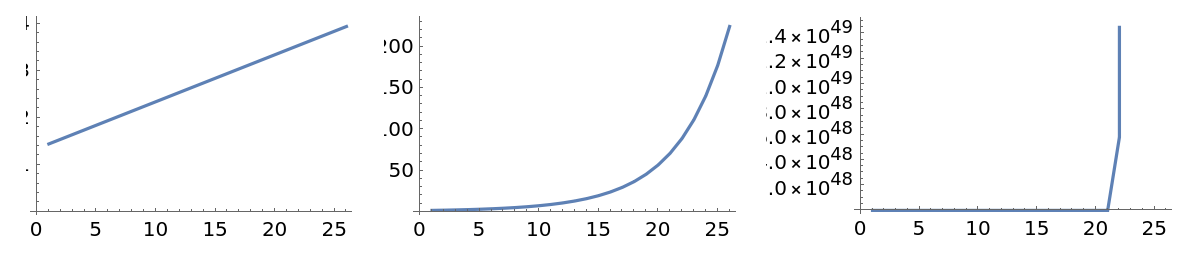The highly divergent property of tetration is easy to see from the previous plots.

### Neat Examples (5)

Real part plot of tetration order 3 for a range of complex values:

 In:=In:=Out=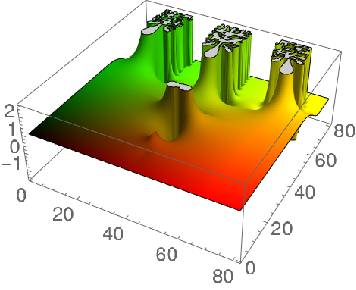Imaginary part plot of tetration order 2:

 In:=Out=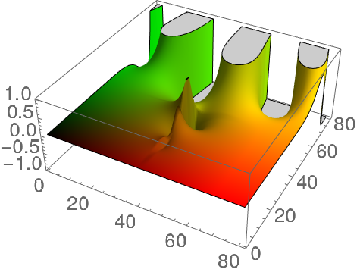Norm plot of tetration order 3:

 In:=Out=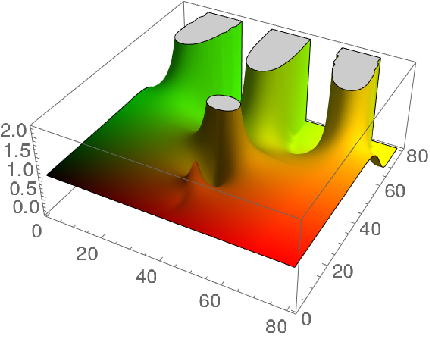Imaginary part of tetration order 2 of a number ez, where z is a complex number:

 In:=Out=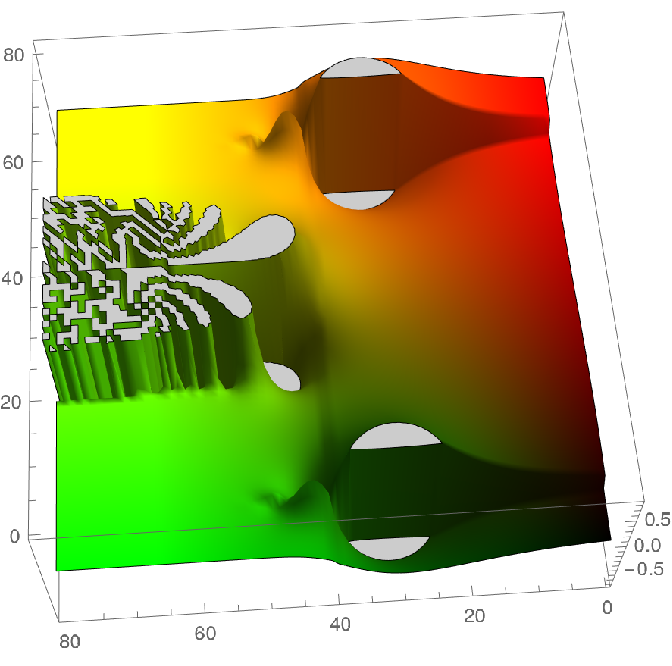Real part of tetration order 2 of a number ez, where z is a complex number:

 In:=Out=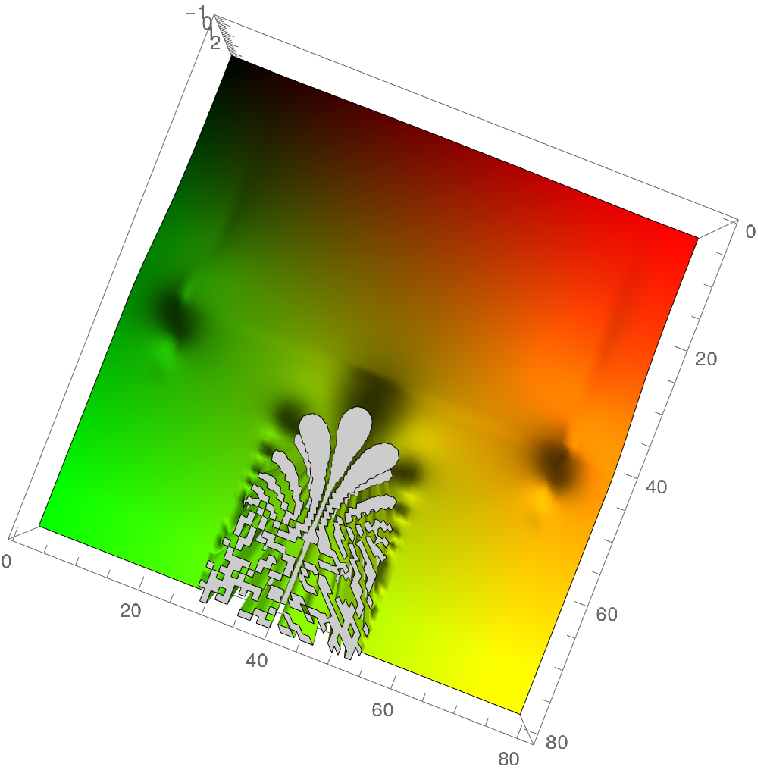### Possible Issues (3)

Tetration of a=0 is Indeterminate:

 In:=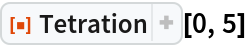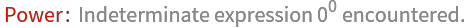Out=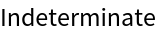Computing tetration for large values of k within the divergence interval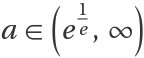may cause overflow, because the tetration exceeded the maximum machine number. You can obtain the maximum k for a given a as such:

 In:=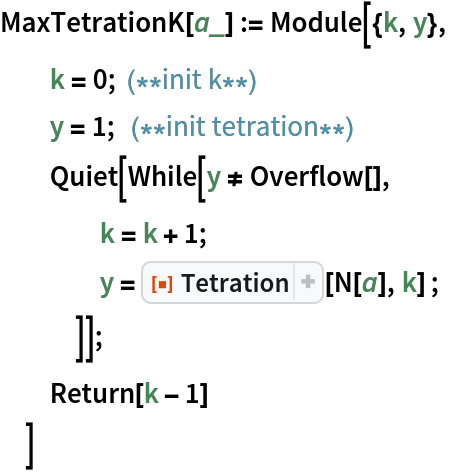Maximum tetration for a=12:

 In:=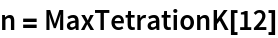Out=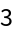If you want to avoid long execution times, use the function N to get an approximated result:

 In:=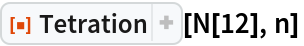Out=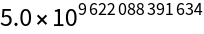Large values of k cause an overflow:

 In:=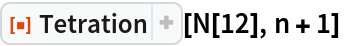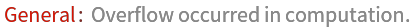Out=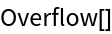Infinite tetration does not exists outside the convergence interval:

 In:=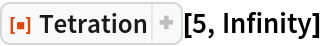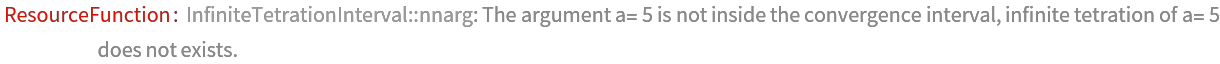## Publisher

WolframSpecialProjects

## Version History

• 1.0.0 – 22 October 2020